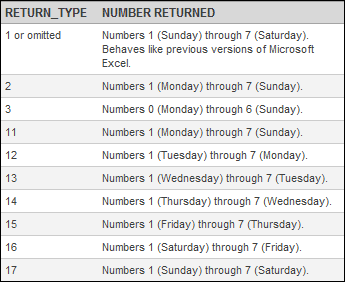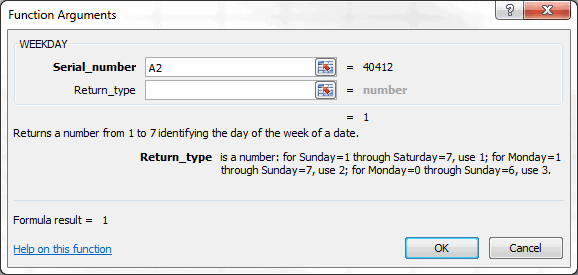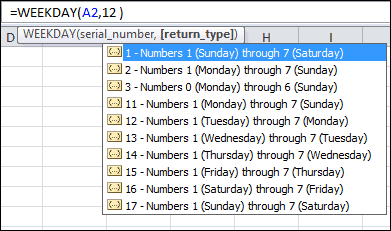# The WEEKDAY Function in ExcelExcel 2010 has new return_type arguments for the WEEKDAY Function. How useful they are is debatable, but you never know when you might come across a particular need.

## Excel Weekday Function

The Excel WEEKDAY Function in has two arguments: serial_number and return_type. The serial_number argument is simply a number that represents a date. The return_type argument is optional, and assumed to be 1 if omitted.

Versions prior to Excel 2010 have only the first three return_type arguments: 1,2 3.  The new arguments are 11-17, representing differing combinations for the starting day of the week.

The Excel WEEKDAY Function returns an integer value 1-7, based upon the day of the week, with the exception of return_type 3 that returns 0-6.

The Excel WEEKDAY Function Arguments dialog box doesn’t show the new arguments.However in Excel 2010, when you enter the WEEKDAY Function into a cell, they are shown as you enter the second argument.## Weekly Reporting and the Excel WEEKDAY Function

I’ve only used the WEEKDAY Function with regards to reporting data, and then only sporadically.

Companies normally have weekly reporting requirements. However, while every week has seven days, there are two important distinctions. First is the start and end days of each week. Some companies use a Sunday-Saturday week, others a Monday-Sunday. These two are the most common, although I came across one company that had a Saturday-Friday reporting week.

The second distinction in weekly reporting is what day is used to represent the entire week. In the USA it’s common to use a week-ending date for weekly reports, while in Europe a week-commencing date is used quite frequently.

I won’t go into specific uses of the WEEKDAY Function for reporting in this post, but the two distinctions mentioned for weekly reporting make it a handy function to know.## 18 thoughts on “The WEEKDAY Function in Excel”

1.Bill Rouse

I’m trying to make a formula that calculates days late on shipped orders without adding Sat & Sun in the calculation. IE: Order Due Date is 12-14-12 and was shipped on
12-17-12. Order is 1 day late.

Thanks, Bill

1.Gregory

Assume Order Due is in cell A2 and the value is 12-14-12. Assume Order Ship is in cell B2 and the value is 12-17-12. The formula for Days Late, which I put in cell C2, is =NETWORKDAYS(A2,B2)-1 and gives the result of 1. The NETWORKDAYS function excludes weekend days. You have to subtract 1 because if the start and end dates are the same the function returns a 1. So if Order Due and Order Ship are both 12/14/12 then the Days Late value is zero.

After adding that formula you normally have to change the cell formatting back to General. You’ll know to change the cell formatting if the answer is 1-1-00.

2.Cathy

Hello,
I have a start date and a completion date which i would like to calculate Monday to Friday days only between the two dates. Start date 18/03/2013 (Cell R2) i would like to calculate Monday to Friday from this date in R2 to be the completion date in cell W2. Please help 🙂

1.Gregory

Monday to Friday days only are weekdays, which are associated with the NETWORKDAYS Function. Per Excel Help: (It) Returns the number of whole working days between start_date and end_date.

=NETWORKDAYS(R2,W2)

3.John

I want to use weekday to work out a shift pattern. Is there a formula where I can just put in the shift pattern and switch the number (week start day) so that I can work out the optimum shift pattern that gives even weekend work days between shifts.

1.Gregory

Check out the Help section of the WEEKDAY function where the second argument can change the week start day for the formula. In later versions of Excel for Windows (2007, 2010, 2013) you can cycle through the entire week for start days. In Excel for Mac you are restricted to the following:

1 or omitted returns numbers 1 (Sunday) through 7 (Saturday). Behaves like earlier versions of Excel.
2 returns numbers 1 (Monday) through 7 (Sunday).
3 returns numbers 0 (Monday) through 6 (Sunday).

4.Mark Rohde

I report sales by normal sales day (M-F) but occasionally get an invoice on weekends which throws off average days sales, etc. I would like to be able to determine if the date is a Sat or Sun and if so make it a Friday (if Sat) or Monday (if Sun) BUT – there’s always a but!- never change the calendar month (so Sat would be Monday if it is the 1st and Sunday would be Friday if Monday is the 1st.) Confused yet?

1.Gregory

Not confuse, but busy. Yet this bugs me so I spent some time to figure it out. Assume you have date in cell A2. Here is the formula you are looking for:

=IF(WEEKDAY(A2,2)<6,A2,IF(WEEKDAY(A2,2)=6,IF(MONTH(A2)=MONTH(A2-1),A2-1,A2+2),IF(WEEKDAY(A2,2)=7,IF(MONTH(A2)=MONTH(A2+1),A2+1,A2-2))))

I tested it and it works beautifully.

5.Bianca

I have list of dates in Column C, I would like Column B to show me the week ending date (Sunday) So I can select the individual week. At present I can only find a formula that gives me the week number rather than a day & date.

1.Gregory

The formula you are looking for is:

=C1-WEEKDAY(C1,2)+7

which will give you a week ending date that is always on Sunday.

1.Bianca

Thank you! This is exactly what I needed. 🙂

2.Bianca

Can I also use a similar formula to show the date as the first of each month so I can group them by month?

1.Gregory

You can use this to show the date of the first day of the month.

=DATE(YEAR(C1),MONTH(C1),1)

6.Sarah

I want to put a schedule together starting on Monday, June 24th, 2013 (column A5), then I want to add one weekday in (A7). I cannot get the formula to work out.

1.Gregory

I don’t know what your formula is, but if you put =A5+1 in cell A7 you will get Tuesday, June 25th, 2013.

7.Sarah

That is correct, but if A% is a Friday, June 28th, and I use the formula =A5+1 in A7, I will get Saturday, June 29th, but I want it to = Monday, July 1st.

8.Mujeeb

Hi All,
1.Gregory